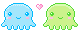Not Logged In
 stay logged in

 A Rose Isn't a Rose Without It's Thornsby †ara
 previous entry: Your Worst... next entry: LOL!!!

# :-D

07/17/201212in12-2011 & Writers Workshop[Private] If: A B C D E F G H I J K L M N O P Q R S T U V W X Y Z is represented as: 1 2 3 4 5 6 7 8 9 10 11 12 13 14 15 16 17 18 19 20 21 22 23 24 25 26. Then: H-A-R-D-W-O-R-K 8+1+18+4+23+15+18+11 = 98% and K-N-O-W-L-E-D-G-E 11+14+15+23+12+5+4+7+5 = 96% But, A-T-T-I-T-U-D-E 1+20+20+9+20+21+4+5 = 100% And, B-U-L-L- S-H-I-T 2+21+12+12+19+8+9+20 = 103% AND, look how far ass kissing will take you. A-S-S-K-I-S-S-I-N-G 1+19+19+11+9+19+19+9+14+7 = 118% So, one can conclude with mathematical certainty that While Hard work and Knowledge will get you close, and Attitude will get you there, it's the bullshit and Ass kissing that will put you over the top.
 previous entry: Your Worst... next entry: LOL!!!

 Add CommentPlease enter the following WHITE digits in the box below.privatelmfao thats awesomeLove,
Jess

[Jessbabyblue|0 likes] [|reply]

LOL This is so true too!Hehehe.[~ Wild One ~|0 likes] [|reply]